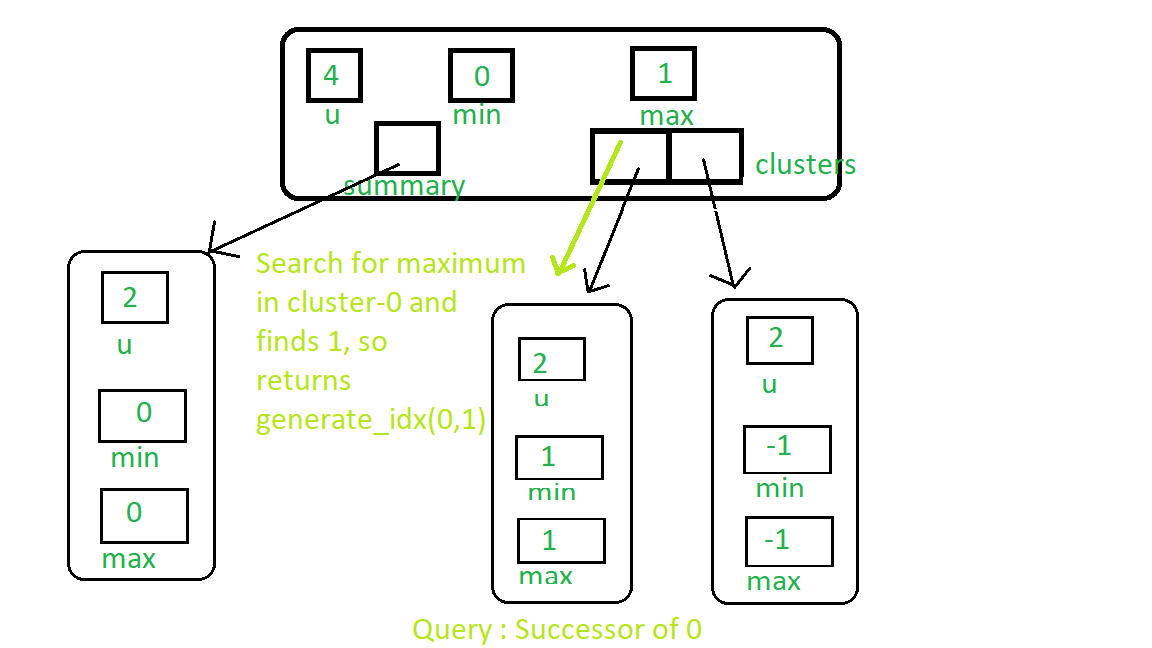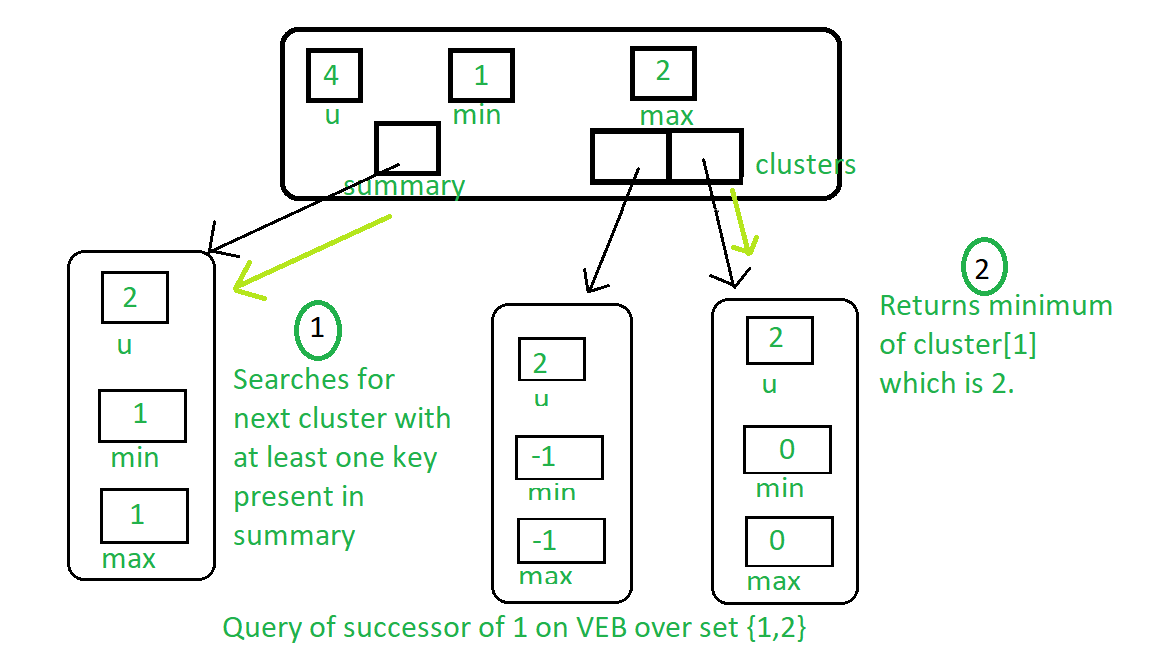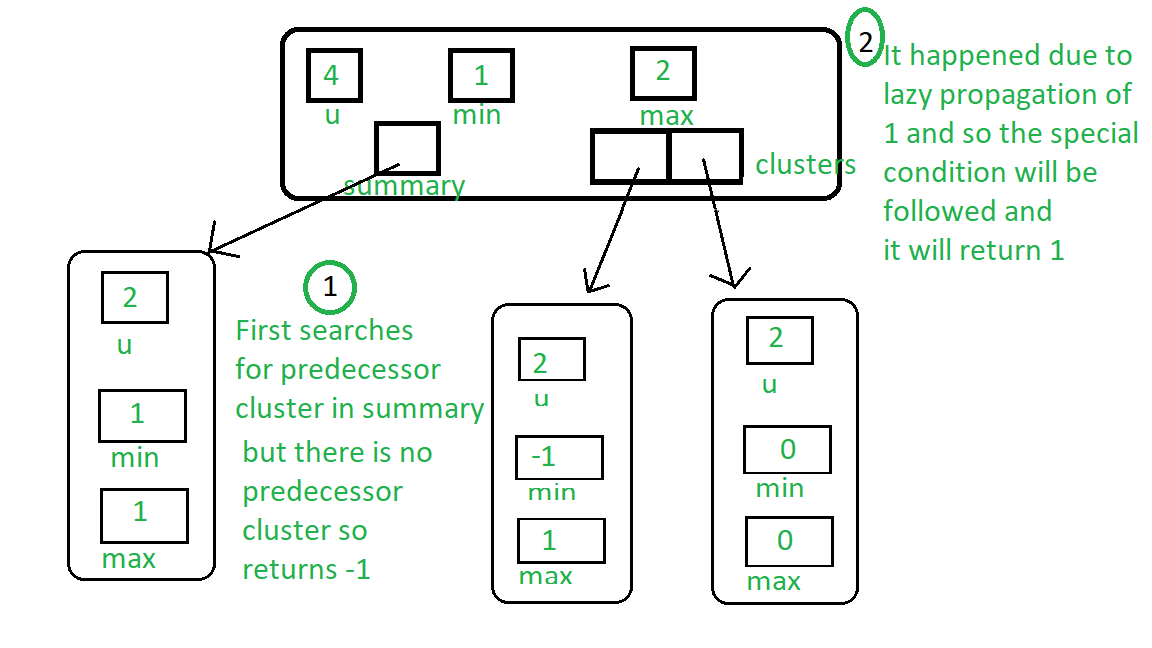Open in App
Not now

# Van Emde Boas Tree – Set 3 | Successor and Predecessor

• Difficulty Level : Hard
• Last Updated : 24 Sep, 2021

It is highly recommended to read the previous articles on Van Emde Boas Tree first.

Procedure for successor:

• Base case: If the size of the tree is 2 then if query-key is 0 and key – 1 is present in the tree then return 1, as it will be the successor. Otherwise, return null.
• If the key is less than minimum then we can easily say that minimum will be the successor of the query-key.
• Recursive case:
• We first search for the successor in the cluster in which the key is present.
• If we find any successor in the cluster then generate its index and return it.
• Otherwise, search for the next cluster, with at least one key present, in summary, and return the index the minimum of that cluster.

See query for the successor of 0 the in below image:The below image represents the successor of 1 query over VEB tree containing key 1 & 2:Procedure for Predecessor:

• Base case: If the size of the tree is 2 then if query-key is 1 and key-0 is present in the tree then return 0, as it will be the predecessor. Otherwise, return null.
• If the key is greater than the maximum then we can easily say that maximum will be the predecessor of the query-key.
• Recursive case:
• We first search for the predecessor in the cluster in which the key is present.
• If we find any predecessor in the cluster then generate its index and return it.
• Otherwise, search for the previous cluster, with at least one key present, in summary. If any cluster is present then return the index of the maximum of that cluster.
• If no cluster with that property is present then see if due to lazy propagation, the minimum of the tree(In which the cluster is present) is less than the key, if yes then return minimum otherwise return null.

Below image represents query predecessor of key-2:## C++

 `#include ``using` `namespace` `std;` `class` `Van_Emde_Boas {` `public``:``    ``int` `universe_size;``    ``int` `minimum;``    ``int` `maximum;``    ``Van_Emde_Boas* summary;``    ``vector clusters;` `    ``// Function to return cluster numbers``    ``// in which key is present``    ``int` `high(``int` `x)``    ``{``        ``int` `div` `= ``ceil``(``sqrt``(universe_size));``        ``return` `x / ``div``;``    ``}` `    ``// Function to return position of x in cluster``    ``int` `low(``int` `x)``    ``{``        ``int` `mod = ``ceil``(``sqrt``(universe_size));``        ``return` `x % mod;``    ``}` `    ``// Function to return the index from``    ``// cluster number and position``    ``int` `generate_index(``int` `x, ``int` `y)``    ``{``        ``int` `ru = ``ceil``(``sqrt``(universe_size));``        ``return` `x * ru + y;``    ``}` `    ``// Constructor``    ``Van_Emde_Boas(``int` `size)``    ``{``        ``universe_size = size;``        ``minimum = -1;``        ``maximum = -1;` `        ``// Base case``        ``if` `(size <= 2) {``            ``summary = nullptr;``            ``clusters = vector(0, nullptr);``        ``}``        ``else` `{``            ``int` `no_clusters = ``ceil``(``sqrt``(size));` `            ``// Assigning VEB(sqrt(u)) to summary``            ``summary = ``new` `Van_Emde_Boas(no_clusters);` `            ``// Creating array of VEB Tree pointers of size sqrt(u)``            ``clusters = vector(no_clusters, nullptr);` `            ``// Assigning VEB(sqrt(u)) to all its clusters``            ``for` `(``int` `i = 0; i < no_clusters; i++) {``                ``clusters[i] = ``new` `Van_Emde_Boas(``ceil``(``sqrt``(size)));``            ``}``        ``}``    ``}``};` `// Function to return the minimum value``// from the tree if it exists``int` `VEB_minimum(Van_Emde_Boas* helper)``{``    ``return` `(helper->minimum == -1 ? -1 : helper->minimum);``}` `// Function to return the maximum value``// from the tree if it exists``int` `VEB_maximum(Van_Emde_Boas* helper)``{``    ``return` `(helper->maximum == -1 ? -1 : helper->maximum);``}` `// Function to insert a key in the tree``void` `insert(Van_Emde_Boas* helper, ``int` `key)``{``    ``// If no key is present in the tree``    ``// then set both minimum and maximum``    ``// to the key (Read the previous article``    ``// for more understanding about it)``    ``if` `(helper->minimum == -1) {``        ``helper->minimum = key;``        ``helper->maximum = key;``    ``}``    ``else` `{``        ``if` `(key < helper->minimum) {` `            ``// If the key is less than the current minimum``            ``// then swap it with the current minimum``            ``// because this minimum is actually``            ``// minimum of one of the internal cluster``            ``// so as we go deeper into the Van Emde Boas``            ``// we need to take that minimum to its real position``            ``// This concept is similar to "Lazy Propagation"``            ``swap(helper->minimum, key);``        ``}` `        ``// Not base case then...``        ``if` `(helper->universe_size > 2) {` `            ``// If no key is present in the cluster then insert key into``            ``// both cluster and summary``            ``if` `(VEB_minimum(helper->clusters[helper->high(key)]) == -1) {``                ``insert(helper->summary, helper->high(key));` `                ``// Sets the minimum and maximum of cluster to the key``                ``// as no other keys are present we will stop at this level``                ``// we are not going deeper into the structure like``                ``// Lazy Propagation``                ``helper->clusters[helper->high(key)]->minimum = helper->low(key);``                ``helper->clusters[helper->high(key)]->maximum = helper->low(key);``            ``}``            ``else` `{``                ``// If there are other elements in the tree then recursively``                ``// go deeper into the structure to set attributes accordingly``                ``insert(helper->clusters[helper->high(key)], helper->low(key));``            ``}``        ``}` `        ``// Sets the key as maximum it is greater than current maximum``        ``if` `(key > helper->maximum) {``            ``helper->maximum = key;``        ``}``    ``}``}` `// Function that returns true if the``// key is present in the tree``bool` `isMember(Van_Emde_Boas* helper, ``int` `key)``{` `    ``// If universe_size is less than the key``    ``// then we can not search the key so returns``    ``// false``    ``if` `(helper->universe_size < key) {``        ``return` `false``;``    ``}` `    ``// If at any point of our traversal``    ``// of the tree if the key is the minimum``    ``// or the maximum of the subtree, then``    ``// the key is present so returns true``    ``if` `(helper->minimum == key || helper->maximum == key) {``        ``return` `true``;``    ``}``    ``else` `{` `        ``// If after attending above condition,``        ``// if the size of the tree is 2 then``        ``// the present key must be``        ``// maximum or minimum of the tree if it``        ``// is not then it returns false because key``        ``// can not be present in the sub tree``        ``if` `(helper->universe_size == 2) {``            ``return` `false``;``        ``}``        ``else` `{` `            ``// Recursive call over the cluster``            ``// in which the key can be present``            ``// and also pass the new position of the key``            ``// i.e., low(key)``            ``return` `isMember(helper->clusters[helper->high(key)],``                            ``helper->low(key));``        ``}``    ``}``}` `// Function to find the successor of the given key``int` `VEB_successor(Van_Emde_Boas* helper, ``int` `key)``{` `    ``// Base case: If key is 0 and its successor``    ``// is present then return 1 else return null``    ``if` `(helper->universe_size == 2) {` `        ``if` `(key == 0 && helper->maximum == 1) {``            ``return` `1;``        ``}``        ``else` `{``            ``return` `-1;``        ``}``    ``}` `    ``// If key is less then minimum then return minimum``    ``// because it will be successor of the key``    ``else` `if` `(helper->minimum != -1 && key < helper->minimum) {` `        ``return` `helper->minimum;``    ``}``    ``else` `{` `        ``// Find successor inside the cluster of the key``        ``// First find the maximum in the cluster``        ``int` `max_incluster = VEB_maximum(helper->clusters[helper->high(key)]);` `        ``int` `offset{ 0 }, succ_cluster{ 0 };` `        ``// If there is any key( maximum!=-1 ) present in the cluster then find``        ``// the successor inside of the cluster``        ``if` `(max_incluster != -1 && helper->low(key) < max_incluster) {` `            ``offset = VEB_successor(helper->clusters[helper->high(key)],``                                   ``helper->low(key));` `            ``return` `helper->generate_index(helper->high(key), offset);``        ``}` `        ``// Otherwise look for the next cluster with at least one key present``        ``else` `{` `            ``succ_cluster = VEB_successor(helper->summary, helper->high(key));` `            ``// If there is no cluster with any key present``            ``// in summary then return null``            ``if` `(succ_cluster == -1) {``                ``return` `-1;``            ``}` `            ``// Find minimum in successor cluster which will``            ``// be the successor of the key``            ``else` `{` `                ``offset = VEB_minimum(helper->clusters[succ_cluster]);` `                ``return` `helper->generate_index(succ_cluster, offset);``            ``}``        ``}``    ``}``}` `// Function to find the predecessor of the given key``int` `VEB_predecessor(Van_Emde_Boas* helper, ``int` `key)``{` `    ``// Base case: If the key is 1 and it's predecessor``    ``// is present then return 0 else return null``    ``if` `(helper->universe_size == 2) {` `        ``if` `(key == 1 && helper->minimum == 0) {``            ``return` `0;``        ``}``        ``else``            ``return` `-1;``    ``}` `    ``// If the key is greater than maximum of the tree then``    ``// return key as it will be the predecessor of the key``    ``else` `if` `(helper->maximum != -1 && key > helper->maximum) {` `        ``return` `helper->maximum;``    ``}``    ``else` `{` `        ``// Find predecessor in the cluster of the key``        ``// First find minimum in the key to check whether any key``        ``// is present in the cluster``        ``int` `min_incluster = VEB_minimum(helper->clusters[helper->high(key)]);` `        ``int` `offset{ 0 }, pred_cluster{ 0 };` `        ``// If any key is present in the cluster then find predecessor in``        ``// the cluster``        ``if` `(min_incluster != -1 && helper->low(key) > min_incluster) {` `            ``offset = VEB_predecessor(helper->clusters[helper->high(key)],``                                     ``helper->low(key));` `            ``return` `helper->generate_index(helper->high(key), offset);``        ``}` `        ``// Otherwise look for predecessor in the summary which``        ``// returns the index of predecessor cluster with any key present``        ``else` `{` `            ``pred_cluster = VEB_predecessor(helper->summary, helper->high(key));` `            ``// If no predecessor cluster then...``            ``if` `(pred_cluster == -1) {` `                ``// Special case which is due to lazy propagation``                ``if` `(helper->minimum != -1 && key > helper->minimum) {``                    ``return` `helper->minimum;``                ``}` `                ``else``                    ``return` `-1;``            ``}` `            ``// Otherwise find maximum in the predecessor cluster``            ``else` `{` `                ``offset = VEB_maximum(helper->clusters[pred_cluster]);` `                ``return` `helper->generate_index(pred_cluster, offset);``            ``}``        ``}``    ``}``}` `// Driver code``int` `main()``{` `    ``Van_Emde_Boas* veb = ``new` `Van_Emde_Boas(8);` `    ``// Inserting Keys``    ``insert(veb, 2);``    ``insert(veb, 3);``    ``insert(veb, 4);``    ``insert(veb, 6);` `    ``// Queries``    ``cout << VEB_successor(veb, 2) << endl;` `    ``cout << VEB_predecessor(veb, 6) << endl;` `    ``cout << VEB_successor(veb, 4) << endl;` `    ``return` `0;``}`

Output:

```3
4
6```

My Personal Notes arrow_drop_up